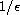Electron. J. Diff. Equ., Vol. 2010(2010), No. 06, pp. 1-19.

### Slow and fast systems with Hamiltonian reduced problems Maamar Benbachir, Karim Yadi, Rachid Bebbouchi

Abstract:
Slow and fast systems are characterized by having some of the derivatives multiplied by a small parameter. We study systems of reduced problems which are Hamiltonian equations, with or without a slowly varying parameter. Tikhonov's theorem gives approximate solutions for times of order 1. Using the stroboscopic method, we give approximations for time of order. More precisely, the variation of the total energy of the problem, and the eventual slow parameter, are approximated by a certain averaged differential equation. The results are illustrated by some numerical simulations. The results are formulated in classical mathematics and proved within internal set theory which is an axiomatic approach to nonstandard analysis.

Submitted June 1, 2009. Published January 13, 2010.
Math Subject Classifications: 34D15, 34E18, 70H09, 03H05.
Key Words: Singular perturbations; Hamiltonian system; stroboscopy lemma; nonstandard analysis.

Show me the PDF file (405 KB), TEX file, and other files for this article.Maamar Benbachir Université de Béchar, BP 417 Route de Kenadsa, 08000 Béchar, Algérie email: mbenbachir2001@yahoo.fr Karim Yadi Laboratoire des Systémes Dynamiques et Applications Université Aboubekr Belkaïd BP 119, 13000 Tlemcen Algérie email: yadikdz@yahoo.fr, k_yadi@mail.univ-tlemcen.dz Rachid Bebbouchi Faculté de Mathématiques Université des Sciences et de la Technologie Houari Boumediéne, Alger Bab ezzouar, Algérie email: rbebbouchi@hotmail.com